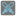• # Army definition

## Question related to missionArmy Battles

Why does the following not work?

```class Army:

units = []

def add_units(self, unit_type, amount, units=units):
for x in range(amount):
units.append(unit_type())
```

I inspected it and verified that it correctly creates the class and correctly adds units. Yet the code doesn't check out. If I instead define the class like this:

```class Army:

def __init__(self):
self.units = []

def add_units(self, unit_type, amount):
for x in range(amount):
self.units.append(unit_type())
```

Then it works. I don't understand why the first piece of code does not. What's the difference between these two?